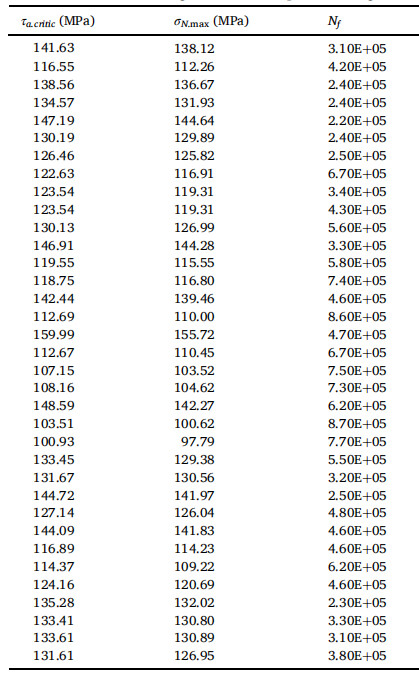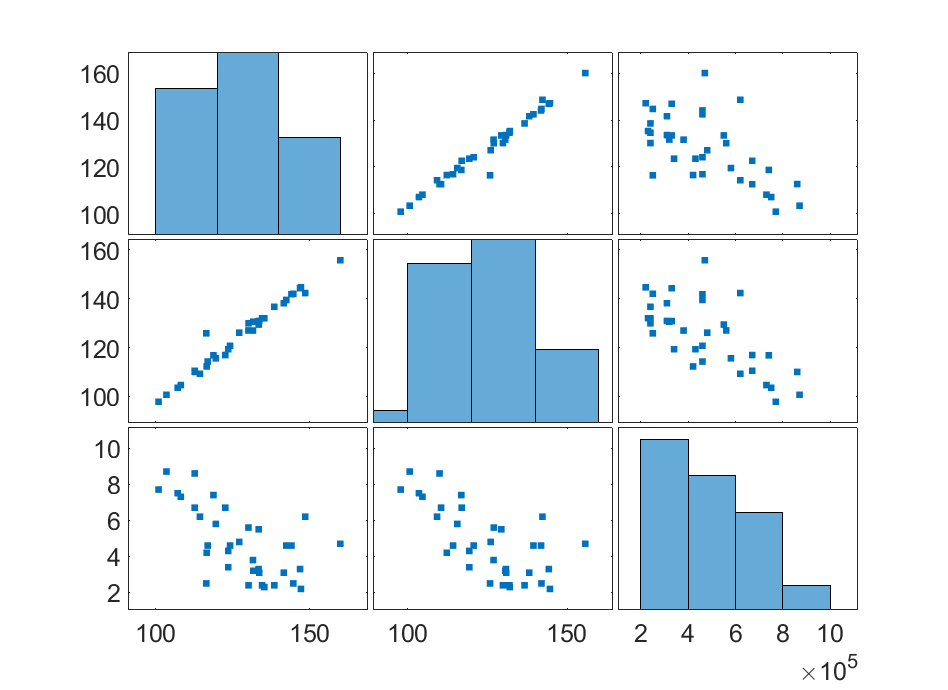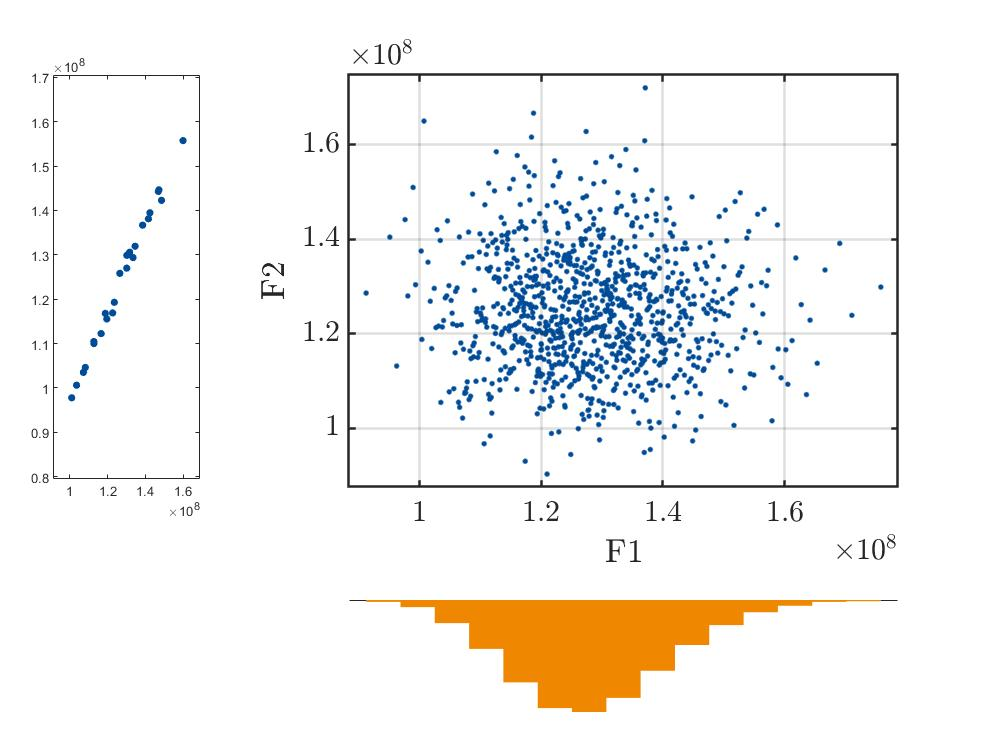# Could we Infer the type of unknown probability distribution of the experimental data?

Dear UQLab，
Many appreciate the sustainable support of UQTeam.

Recently I am stuck with a problem：
I want to construct a high-precision PCE model of the data in the following figure ,the first two columns are the inputs and the third column is the output, the first 25 sets as DOE and the last 10 sets as the validation setBut I don’t know what kind of distribution the data follow and what the mean value of the distribution is.？

I tried to use the statistical inference tool in the INPUT module to infer the type of distribution they follow, but the accuracy of the constructed PCE model is still not satisfactory (best LOO > 0.3)

I am not sure what could be the reason for this, could it be that the data itself is not suitable for metamodeling?

Thanks again to UQLab, a milestone tool that has helped me tremendously！

bests

Dear Felix

As a general advice, you should always plot your dataset using `plotmatrix` first. In your case, we get the image below:You see that the two variables X_1 and X_2 are almost perfectly correlated. Moreover, the third parameter (what you call output) is loosely correlated to the first ones. In other words, there is no deterministic mapping between (X_1,X_2) and Y. You cannot apply PCE (nor any other standard surrogate) in this context.

Best regards
Bruno

1 Like

Dear Prof.
I understand the reason for the error, and thank you very much for your valuable advice.
Thanks again!

bests

Dear Prof.
Thank you very much for the advice on plotmatrix, but I couldn’t find the usage for this handle in the user of INPUT and Statistical inference module.

I can only analyze the correlation between X1/X2/Y step by step by trying to draw the following diagram

``````%% 3 - INPUT MODEL
InputOpts.Marginals(1).Name = 'F1';
InputOpts.Marginals(1).Type = 'Lognormal'; %Uniform\Gaussian\Lognormal\Gumbel
InputOpts.Marginals(1).Moments = [128.192e6 12.8192e6];

InputOpts.Marginals(2).Name = 'F2';
InputOpts.Marginals(2).Type = 'Lognormal' ;
InputOpts.Marginals(2).Moments = [125.072e6 12.5072e6];
%%
myInput = uq_createInput(InputOpts);
uq_display(myInput);
uq_display(myInput, [1,2]);
%%
X1=X(:,1);
X2=X(:,2);
subplot(1,3,1);
plotmatrix(X1,X2);
subplot(1,3,2);
plotmatrix(X1,Y);
subplot(1,3,3);
plotmatrix(X2,Y);
``````Is there a handle in Uqlab that draws the legend directly from your answer?
Many thanks

Bests